### Communities

tag:snake search within a tag
user:xxxx search by author id
score:0.5 posts with 0.5+ score
"snake oil" exact phrase
created:<1w created < 1 week ago
post_type:xxxx type of post
Challenges

# Label a hinged tetromino

+3
−0

Given a hinged tetromino, give it a unique, consistent label independent of location, rotation, and reflection.

## Tetrominoes

A tetromino is a connected subset of the square tiling, composed of 4 squares, where connection can only be edge connection.

Here are the 5 tetrominoes:## Hinged tetrominoes

A hinged tetromino is a connected subset of the square tiling, composed of 4 squares, where connection can be edge connection or corner connection.

Here are the 22 hinged tetrominoes:## Equivalence

The following 8 shapes are all considered to be the same hinged tetromino. They are equivalent because any one can be reached from any other by some combination of rotation, reflection, and translation:All 8 of these rotated and reflected versions must have the same label. Translating, rotating, or reflecting a hinged tetromino must not change its label.

## Input

• A 4 by 4 grid containing a hinged tetromino.
• Apart from the 4 squares of the hinged tetromino, all other squares are empty.
• You may take input in any convenient format that does not include full or partial precalculation. For example:
• A string or sequence of strings.
• A 1 or 2 dimensional list/array/vector.
• A sequence of coordinates (even a sequence of X coordinates and a separate sequence of Y coordinates if you wish).
• A 16 bit variable where each 1 bit represents part of the hinged tetromino and each 0 bit represents part of the background.
• A variable with more than 16 bits where only 16 of the bits are used would also be acceptable.

## Output

• A label for the hinged tetromino.
• The label for each hinged tetromino can be anything you choose, including nothing (no output).
• Each label must be unique (it must not be the label for any other hinged tetromino).
• Each label must be consistent (a given hinged tetromino must always result in the same label, regardless of rotation, reflection, or translation).

## Scoring

This is a code golf challenge. Your score is the number of bytes in your code.

Explanations are optional, but I'm more likely to upvote answers that have one.

Why does this post require moderator attention?
Why should this post be closed?

Clarification on rotations, etc. (2 comments)

+1
−0

# JavaScript (Node.js), 892 bytes (not fully working)

I=>{N=a=>Math.min(...a);X=a=>Math.max(...a)
C=[];s=[t=[],[]];S="splice";p="push"
c=I[P="map"](a=>a[P](p=>p-N(a)))
d=X(c)-X(c[r=0])
d>0?r=1:d||c[P]((a,b)=>a-1||C[p](c[i=0][b]))
if(''+c.filter((a,b)=>C[O="indexOf"](a)>=0&&c[b]!=1)){r=1,C=[],c[P]((a,b)=>a==1&&C[p](c[b]))
if(''+c.filter((a,b)=>C[O](a)>=0&&c[b]!=1)&&X(c)==2)
return c[P]((a,b)=>a-1?c[b]!=1?1:0:c[b]!=1?0:2).reduce((a,b)=>a+b)}
if(r)while(''+c){if(c[i]==N(c))c[P]((a,b)=>{s[1-b].push(a[i]),c[b][S](i,1)}),i=-1;i++}''+s?0:s=c
m=X([1111*X(s)-s[R="reverse"]()[J="join"],+s[R]()[J]])
u=(''+s).split,[P](a=>X(s)-a)
v=(''+s).split,[P](a=>+a)
w=[]
if(m-s[J])while(''+s){if(s[i]==X(s))t[p](s[i]),s[P](a=>a[S](i,1)),i=-1;i++}i=3
while(''+u){if(v[i]==X(v))w[p](u[i]),u[S](i,1),v[S](i,1),i=v.length;i--}''+t?0:t=s
m*=X([+t[J],+w[J]]);return m}


Attempt This Online! (Add input in the footer)

I can confirm that solving something hard, even if it took 6 million years, is very rewarding :) (Though the code's REALLY long)

Definitely can still be optimized, there's no way 892 bytes is the limit.

### How does it work? (Assuming it works, which it doesn't fully yet)

Since symmetry is important, my first thought involved finding the average X- and Y-coordinates of the squares. It works, but sadly the labels occur more than once (meaning they're not unique).

After days of brainstorming on how to make them unique which almost drove me insane, I came to the conclusion we need a better method.

Ah! Here's the better method.

• Let's say the top left grid is [0,0], the X-axis goes left to right, and the Y-axis up to down.
• We separate the X- and Y-coordinates, and mush them each together to form 2 numbers.
• To ensure uniqueness, we then multiply those numbers and assign it as the label. Yeay!

For a better picture, let's label the coordinates of the 10th-13th tetrominoes: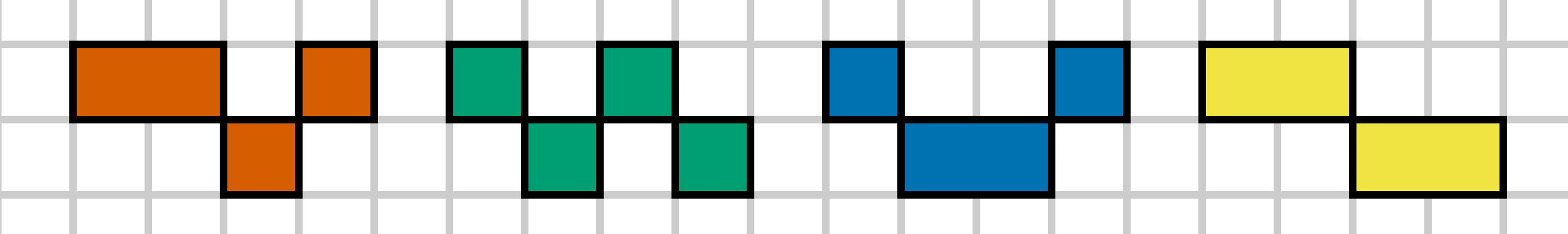We get

[[0,0], [1,0], [3,0], [2,1]],
[[0,0], [2,0], [1,1], [3,1]],
[[0,0], [3,0], [1,1], [2,1]],
[[0,0], [1,0], [2,1], [3,1]].


(Note that the order of the coordinates is just like reading text in most languages (top to bottom, left to right))

Now, mushing the X- and Y-coordinates would look like this:

X: [0,1,3,2] Y: [0,0,0,1]
X: [0,2,1,3] Y: [0,0,1,1]
X: [0,3,1,2] Y: [0,0,1,1]
X: [0,1,2,3] Y: [0,0,1,1]


(Also note that order really, really matters here)

Anyway, when we flip the tetrominoes upside-down: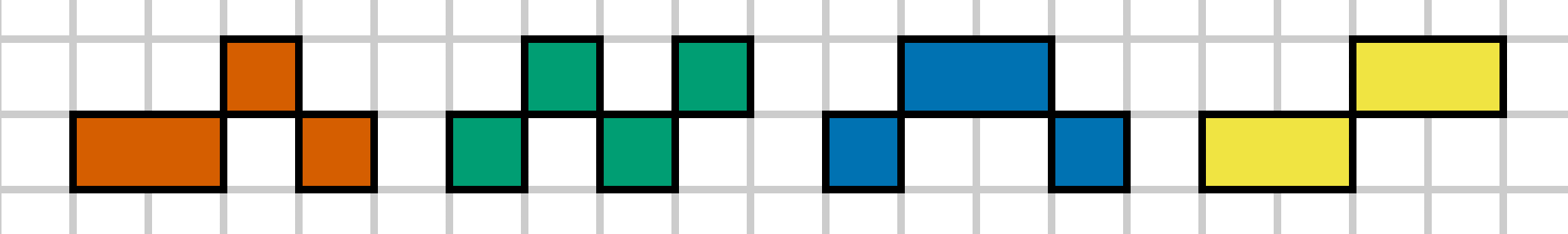The coordinates become

[[2,0], [0,1], [1,1], [3,1]],
[[1,0], [3,0], [0,1], [2,1]],
[[1,0], [2,0], [0,1], [3,1]],
[[2,0], [3,0], [0,1], [1,1]].


What did we achieve? As it turns out, we can flip a tetromino by flipping its Y-coordinates and then rearranging them!

(In this example, we flip the Y-coordinates from 0 to 1 and vice versa. The idea is pretty similar for other tetrominoes.)

Also, notice that the Y-coordinates are always in ascending order, which is what we'll need to do when rearranging.

The same is true when flipping the tetrominoes horizontally: flip the X-coordinates, and rearrange. (Feel free to check this by hand)

Anyway, this means we can get the flipped version of any tetromino. Progress!

What to do next?

• From those flipped tetrominoes, get their mushed together X- and Y-coordinates.
• Compare them to the original tetromino. Use the one with the greatest value.
(That means if we have [0,1,3,2] and [2,0,1,3], we pick the latter because 132 < 2013. So, we get the same result even if we flip the tetrominoes around!)

### Rotating

So far we've solved mirroring (or flipping), but what about the pesky rotation? How do we rotate, and how do we know when to rotate?

My idea is that if a tetromino has two squares side-by-side or is more tall than wide, we rotate. Otherwise, we don't.

For not square-ish tetrominoes like 10-13 (the ones I showed you before), this works well!

However, how about the square-ish ones where its width is the same as its height?

Let's take a look at this guy, the 16th tetromino: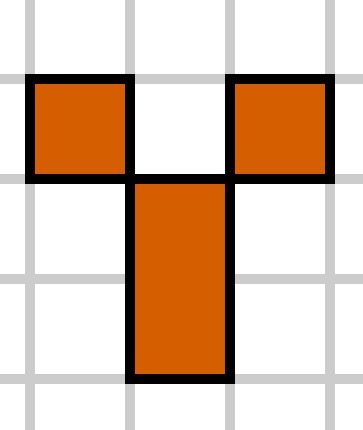Let's call it Yancy. Why not?

...anyway, Yancy is a square-ish 3x3 tetromino, and these are quite annoying (no offense, Yancy).

Yancy here does not have two squares side-by-side. This means we shouldn't rotate it, and it's fine just the way it is :)

However, take Yancy's cousin Yandy (who's quite odd):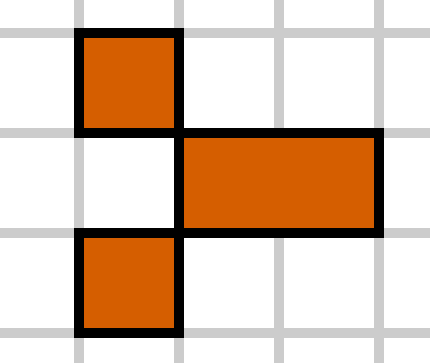Since Yandy has two squares side-by-side, we need to rotate it. The question is how?

Well... after some pondering, here's what I figured out:

• We can think of rotation as a diagonal mirror, something like this: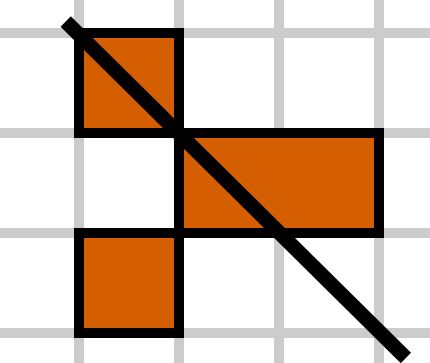(Note that even if the result is flipped, that's still the same tetromino which means it doesn't matter!)
• When we mirror something (like in the image), its X-coordinates become its Y-coordinates and vice versa.
• The only thing left to do is to rearrange them, and we're done!

Now, only a bunch of tetrominoes will always have two blocks side-by-side even when rotated, and they're all symmetric so they definitely won't cause any problems.

Oh wait...

Actually, they do. Thankfully enough, there's an unelegant "hardcoded" way to give them unique labels (see line 6-8 in my code) and I don't have to spend another 6 million years trying to fix that 🙂

After all this, though, I can only hope my effort isn't ruined by a tetromino which I haven't tested on and somehow breaks the code. Feel free to test the code yourself.

Update: I tested every possible transformation, and there were errors in tetrominoes 4-9, 11, 13, 21, and 22. Sigh.

Also, the code's really golfed that not even I can immediately understand it. That's why I didn't bother trying to break down the code :)

Why does this post require moderator attention?

Tested - looks good (5 comments)
+1
−0

# Dyalog APL, 89 bytes

{1∊∘∊¨⍷¨∘⍵¨⍬{0∊⍴⍵:⍺⋄(⍺,⊂A)∇⍵~A←(⌽¨,⊢)(⊖¨,⊢)(⍉¨,⊢)⊣/⍵}(e⍤⍉e←{⍵/⍨×+⌿⍵})¨4 4∘⍴¨,⌿2⊥⍣¯1⍳2*16}


Requires IO to be zero.

Ungolfed version:

{
removeEmptyRows←{⍵/⍨×+⌿⍵}
removeEmptyCols←removeEmptyRows⍉ ⍝ Transpose, then remove the empty rows
dihedral4←(⌽¨,⊢)⍤(⊖¨,⊢)⍤(⍉¨,⊢)⍤⊂ ⍝ All rotations + reflections of a matrix:
⍝ this maps A to the matrices A, ⌽A, ⊖A, ⌽⊖A, ⍉A, ⍉⌽A, ⍉⊖A, ⍉⌽⊖A
⍝ which is equivalent to the classic four rotations and a reflection construction
all4x4Matrices←4 4∘⍴¨,⌿2⊥⍣¯1⍳2*16 ⍝ For every number from 1 to 2*16,
⍝ take the first 16 bits and reshape them into a 4x4 matrix
groupMatrices←⍬∘{   ⍝ Takes 4x4 matrices and builds groups out of their D4's
0∊⍴⍵:⍺            ⍝ If there are no matrices to process, we are done, return the accumulator left argument
mats←dihedral4 ⊃⍵ ⍝ D4 the first matrix in the list
(⍺,⊂mats)∇⍵~mats  ⍝ Recursively call groupMatrices, storing mats in the accumulator and
⍝ removing all elements of mats from the list
}
hasSubmats←1∘∊∘∊¨⍷¨∘⍵¨ ⍝ 1 if any of the argument's matrices appears in the input to the function
hasSubmats groupMatrices removeEmptyCols¨removeEmptyRows¨all4x4Matrices
}


This code can actually uniquely label all subsets of 4x4 grid that have 4 elements, no matter if they satisfy the condition of hinged tetrominos or not. While the code returns an unique sequence of 6110 boolean values for every "sparse tetromino", this table lists instead the indices (0-based) where the list has a 1 (and 0s everywhere else).

┌────┬────────────────┐
│○○○○│0 1 2 3 4       │
├────┼────────────────┤
│○○○ │0 1 2 3 6 8     │
│○   │                │
├────┼────────────────┤
│ ○○○│0 1 2 3 5 7 9   │
│○   │                │
├────┼────────────────┤
│○○○ │0 1 2 3 6 12    │
│ ○  │                │
├────┼────────────────┤
│○ ○○│0 1 2 5 7 11 13 │
│ ○  │                │
├────┼────────────────┤
│○○  │0 1 2 15        │
│○○  │                │
├────┼────────────────┤
│○ ○ │0 1 2 5 6 16    │
│○○  │                │
├────┼────────────────┤
│ ○○ │0 1 2 6 17      │
│○○  │                │
├────┼────────────────┤
│  ○○│0 1 2 5 7 19    │
│○○  │                │
├────┼────────────────┤
│ ○ ○│0 1 5 11 24     │
│○ ○ │                │
├────┼────────────────┤
│○  ○│0 1 2 5 7 28    │
│ ○○ │                │
├────┼────────────────┤
│ ○○ │0 1 2 5 7 41    │
│○   │                │
│○   │                │
├────┼────────────────┤
│○ ○ │0 1 5 11 46     │
│ ○  │                │
│○   │                │
├────┼────────────────┤
│ ○○ │0 1 2 5 6 7 47  │
│ ○  │                │
│○   │                │
├────┼────────────────┤
│  ○○│0 1 2 5 7 45 49 │
│ ○  │                │
│○   │                │
├────┼────────────────┤
│○○  │0 1 2 5 7 60    │
│  ○ │                │
│○   │                │
├────┼────────────────┤
│ ○○ │0 1 2 6 61      │
│  ○ │                │
│○   │                │
├────┼────────────────┤
│ ○ ○│0 1 5 11 59 63  │
│  ○ │                │
│○   │                │
├────┼────────────────┤
│ ○  │0 1 2 5 7 11 67 │
│○ ○ │                │
│○   │                │
├────┼────────────────┤
│○   │0 1 2 5 7 11 75 │
│ ○○ │                │
│○   │                │
├────┼────────────────┤
│ ○  │0 1 2 5 6 7 76  │
│ ○○ │                │
│○   │                │
├────┼────────────────┤
│   ○│0 1 2 5 7 81    │
│ ○○ │                │
│○   │                │
├────┼────────────────┤
│ ○○ │0 1 2 5 7 104   │
│   ○│                │
│○   │                │
├────┼────────────────┤
│  ○ │0 1 5 11 45 112 │
│ ○ ○│                │
│○   │                │
├────┼────────────────┤
│ ○  │0 1 2 5 7 59 128│
│  ○○│                │
│○   │                │
├────┼────────────────┤
│  ○○│0 1 2 5 59 173  │
│○   │                │
│ ○  │                │
├────┼────────────────┤
│○  ○│0 1 5 45 59 183 │
│  ○ │                │
│ ○  │                │
├────┼────────────────┤
│ ○  │0 1 5 11 187    │
│○ ○ │                │
│ ○  │                │
├────┼────────────────┤
│○ ○ │0 1 5 59 213    │
│   ○│                │
│ ○  │                │
├────┼────────────────┤
│  ○ │0 1 5 59 217    │
│○  ○│                │
│ ○  │                │
├────┼────────────────┤
│   ○│0 1 5 45 773    │
│  ○ │                │
│ ○  │                │
│○   │                │
├────┼────────────────┤
│  ○ │0 1 5 59 801    │
│   ○│                │
│ ○  │                │
│○   │                │
├────┼────────────────┤
│   ○│0 1 5 59 1000   │
│ ○  │                │
│  ○ │                │
│○   │                │
├────┼────────────────┤
│ ○  │0 1 5 59 1038   │
│   ○│                │
│  ○ │                │
│○   │                │
├────┼────────────────┤
│ ○  │0 1 5 45 1537   │
│  ○ │                │
│   ○│                │
│○   │                │
├────┼────────────────┤
│  ○ │0 1 5 2765      │
│   ○│                │
│○   │                │
│ ○  │                │
├────┼────────────────┤
│  ○ │0 1 3180        │
│○   │                │
│   ○│                │
│ ○  │                │
└────┴────────────────┘

Why does this post require moderator attention?